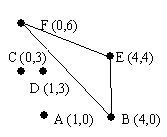시간 제한메모리 제한제출정답맞힌 사람정답 비율
1 초 128 MB17151285.714%

## 문제There has been considerable archeological work on the ancient Myacm culture. Many artifacts have been found in what have been called power fields: a fairly small area, less than 100 meters square where there are from four to fifteen tall monuments with crystals on top. Such an area is mapped out above. Most of the artifacts discovered have come from inside a triangular area between just three of the monuments, now called the power triangle. After considerable analysis archeologists agree how this triangle is selected from all the triangles with three monuments as vertices: it is the triangle with the largest possible area that does not contain any other monuments inside the triangle or on an edge of the triangle. Each field contains only one such triangle.

Archeological teams are continuing to find more power fields. They would like to automate the task of locating the power triangles in power fields. Write a program that takes the positions of the monuments in any number of power fields as input and determines the power triangle for each power field.

A useful formula: the area of a triangle with vertices (x1, y1), (x2, y2), and (x3, y3) is the absolute value of

0.5 × [(y3 - y1)(x2 - x1) - (y2 - y1)(x3 - x1)].

## 입력

For each power field there are several lines of data. The first line is the number of monuments: at least 4, and at most 15. For each monument there is a data line that starts with a one character label for the monument and is followed by the coordinates of the monument, which are nonnegative integers less than 100. The first label is A, and the next is B, and so on.

There is at least one such power field described. The end of input is indicated by a 0 for the number of monuments. The first sample data below corresponds to the diagram in the problem.

## 출력

For each power field there is one line of output. It contains the three labels of the vertices of the power triangle, listed in increasing alphabetical order, with no spaces.

## 예제 입력 1

6
A 1 0
B 4 0
C 0 3
D 1 3
E 4 4
F 0 6
4
A 0 0
B 1 0
C 99 0
D 99 99
0


## 예제 출력 1

BEF
BCD